# Graspable Math

A revisit to Graspable Math.

Graspable Math offers a highly innovative interface for mathematical notation. You can read the Graspable Math story here.

You can learn a great deal about Graspable Math simply by experimenting, you can also find plenty of help and tutorials on the Learn section of the site, note the Gesture Library as well as the video tutorial collection.  There is a YouTube channel here.

To experiment with the interface select Explore algebra online.Graspable Math is easy to use, I decided I would solve an equation and wanted to show all the steps. To start, go to a blank canvas and choose Insert / Math Expression, I have used the method of selecting and holding the = sign to start as you can see illustrated in the video above; I was then able to enter an operation to apply to both sides of the equation.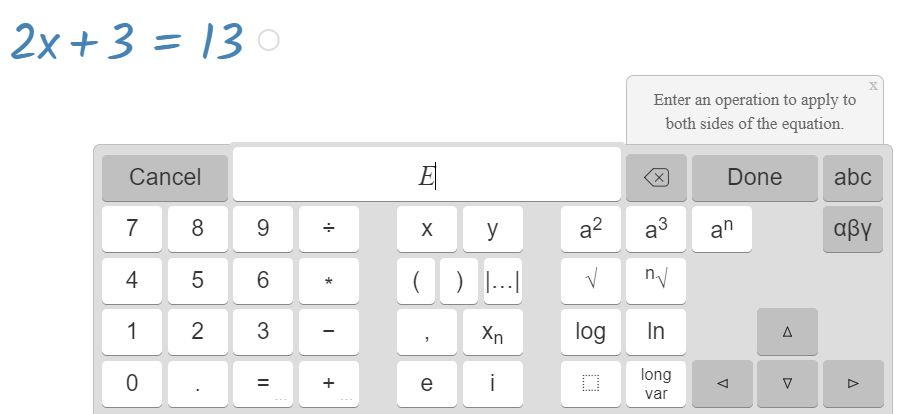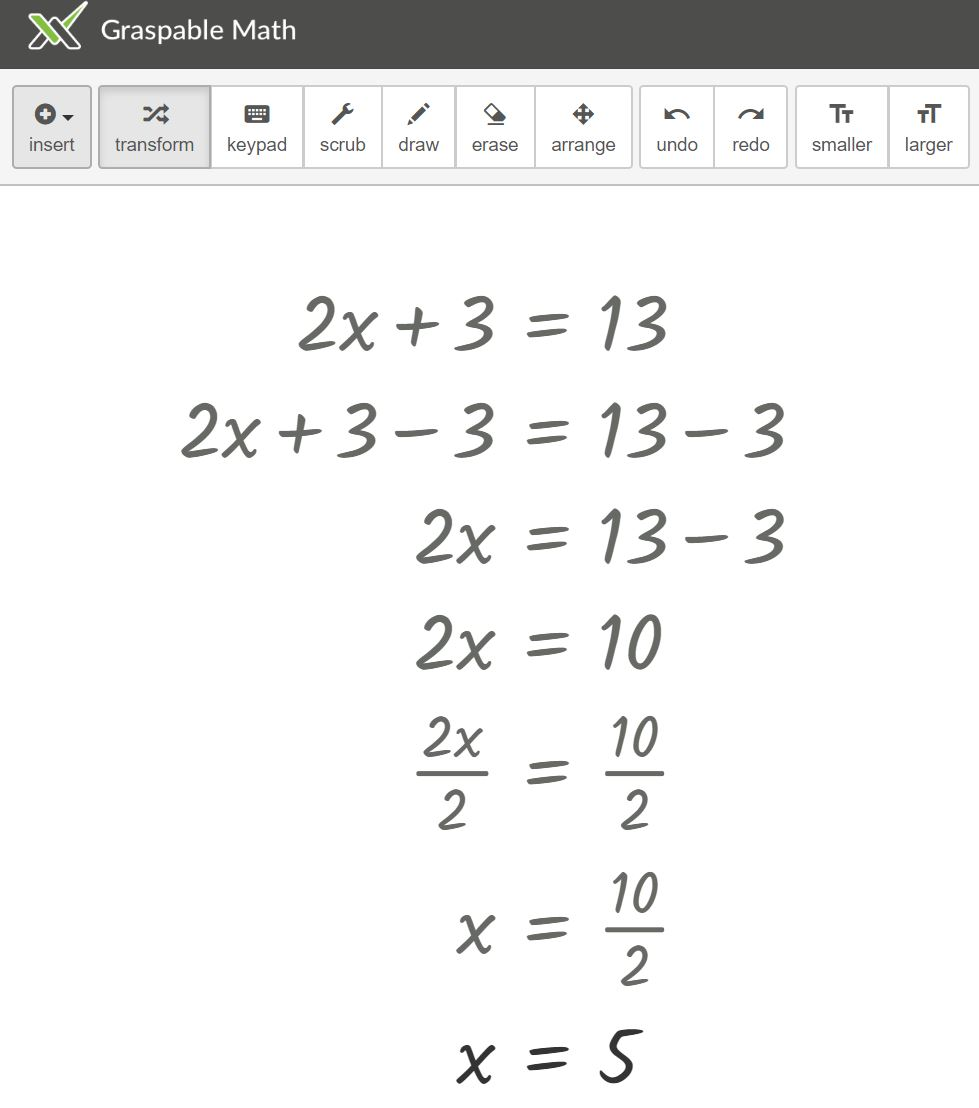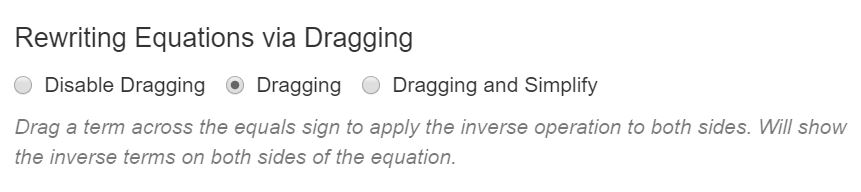An alternative to the above is to use the settings menu; choose Dragging to apply the inverse operation to both sides.

We can also illustrate a solution graphically by inserting a graph to open a GeoGebra window.Each expression has a circle at the end – simply drag that to the GeoGebra window. You will sometimes see more than one circle at the end of an expression, select to separate expressions hence showing all steps clearly.

The scrubbing feature is very useful, drag up or down on a number to change its value; the change will propagate through the rest of the working.A great example of the use of the scrubbing feature can be found on this canvas created by Graspable Math for this lovely problem, Create a System of Two Equations by Daniel Luevanos on Open Middle, accessible for students yet such a great task for mathematical thinking. We could discuss inequalities here as well as simultaneous equations.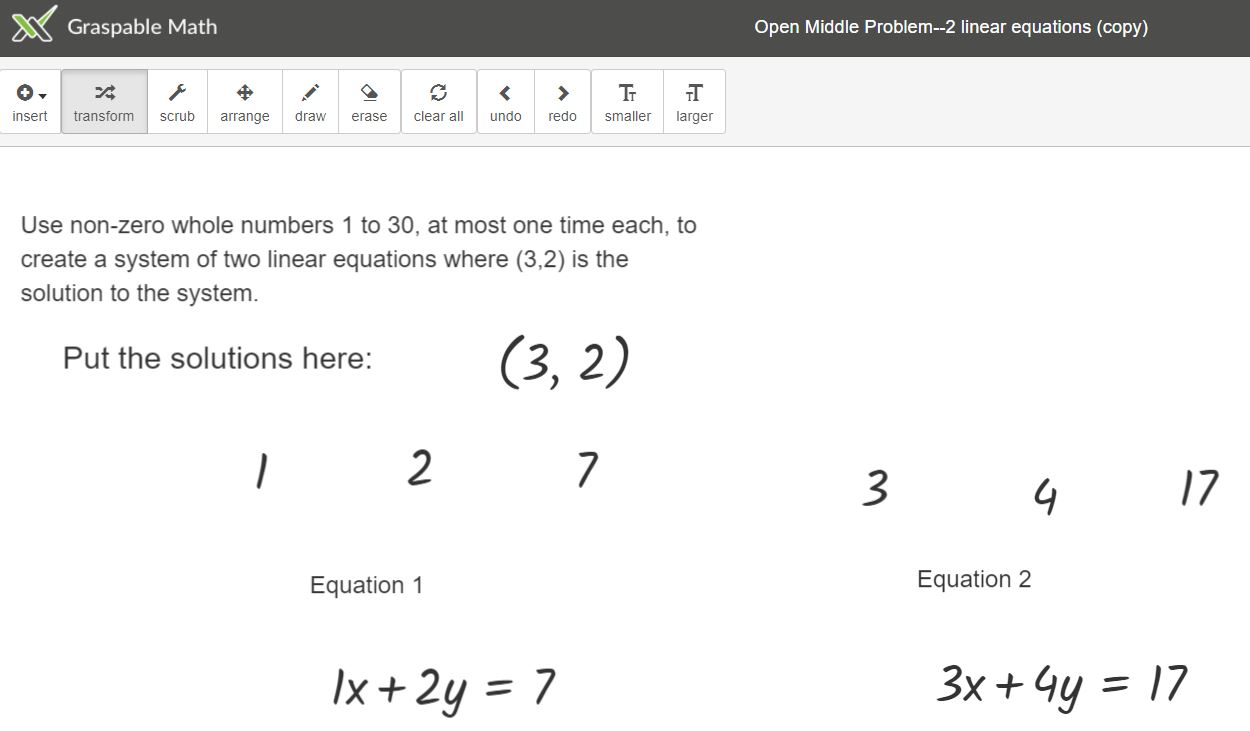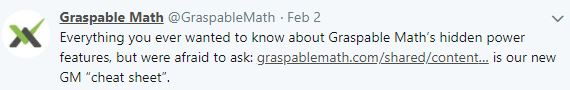Graspable Maths have provided a ‘cheat sheet‘ providing a handy summary of how to use the interface.

Graspable Math took one of Dave Taylor’s wonderful Increasingly Difficult Question sets and created a canvas of the problems.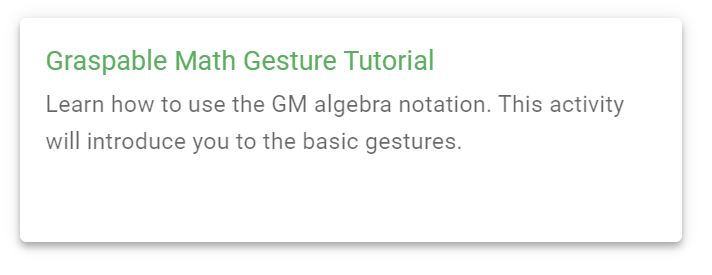We now have Graspable Math Activities, Graspable Math have a free version and optional subscription (watch for information from Graspable Math on this, but teachers can be reassured that they can continue to use Graspable Math with their students). You can use the Activity Bank to learn how to use Graspable Math. Select Graspable Math Gesture Tutorial and you can watch demonstrations and try the features for yourself. This seems a really good way to learn to use the interface.

It is possible to insert a video onto a canvas, so you could inset a video and then follow the instructions on the canvas. Try this on solving equations.

A lovely way to practice – use this One Incorrect canvas by Eric Weitnauer which is based on Don Steward’s one incorrect simplification.Also a good way to learn to use Graspable Math – save a canvas and adapt it, I made a copy of Eric’s canvas and created one for the first problem from Don Steward’s blog post, discovering along the way that if you enter text for a web link, then Graspable Math inserts that as a link.